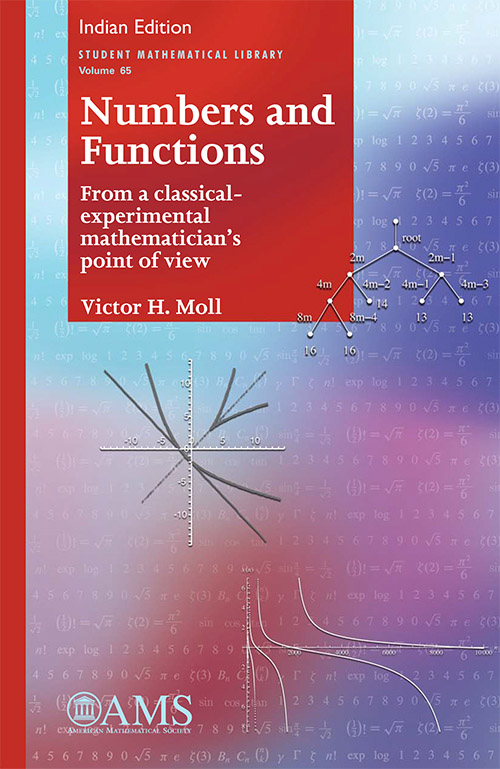Numbers and Functions: From a Classical–Experimental Mathematician's Point of View
Victor H Moll
Price
1740.00
ISBN
9781470425968
Language
English
Pages
504
Format
Paperback
Dimensions
216 x 280 mm
Year of Publishing
2016
Territorial Rights
Restricted
Imprint
Universities Press
Catalogues

New mathematics often comes about by probing what is already known. Mathematicians will change the parameters in a familiar calculation or explore the essential ingredients of a classic proof. Almost magically, new ideas emerge from this process. This book examines elementary functions, such as those encountered in calculus courses, from this point of view of experimental mathematics. The focus is on exploring the connections between these functions and topics in number theory and combinatorics. There is also an emphasis throughout the book on how current mathematical software can be used to discover and prove interesting properties of these functions. The book provides a transition between elementary mathematics and more advanced topics, trying to make this transition as smooth as possible. Many topics occur in the book, but they are all part of a bigger picture of mathematics. By delving into a variety of them, the reader will develop this broad view. The large collection of problems is an essential part of the book. The problems vary from routine verifications of facts used in the text to the exploration of open questions.

Victor H Moll is Professor at the Department of Mathematics, Tulane University, New Orleans, USA.

Preface
Chapter 1. The Number Systems
Chapter 2. Factorials and Binomial Coefficients
Chapter 3. The Fibonacci Numbers
Chapter 4. Polynomials
Chapter 5. Binomial Sums
Chapter 6. Catalan Numbers
Chapter 7. The Stirling Numbers of the Second Kind
Chapter 8. Rational Functions
Chapter 9. Wallis’ Formula
Chapter 10. Farey Fractions
Chapter 11. The Exponential Function
Chapter 12. Trigonometric Functions
Chapter 13. Bernoulli Polynomials
Chapter 14. A Sample of Classical Polynomials: Legendre, Chebyshev, and Hermite
Chapter 15. Landen Transformations
Chapter 16. Three Special Functions: G, ?, and ?
Bibliography
Index

THE BOOKPOINT (INDIA) PVT. LTD.